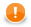### OnError() Functions

Alternatively to the Try-Catch Statement introduced in CloverDX 5.6, you can use a set of optional `OnError()` functions that exist to each required transformation function.

For example, for required functions (e.g. `append()`, `transform()`, etc.), there exist following optional functions:

`appendOnError()`, `transformOnError()`, etc.

Each of these required functions may have its (optional) counterpart whose name differs from the original (required) by adding the `OnError` suffix.

Moreover, every `<required ctl template function>OnError()` function returns the same values as the original required function.

This way, any exception that is thrown by the original required function causes call of its `<required ctl template function>OnError()` counterpart (e.g. `transform()` fail may call `transformOnError()`, etc.).

In this `transformOnError()`, any incorrect code can be fixed, an error message can be printed to the Console, etc.

ImportantRemember that these `OnError()` functions are not called when the original required functions return Error codes (values less then -1). If you want some `OnError()` function to be called, you need to use the `raiseError(string arg)` function. Or (as stated before) any exception thrown by original required function calls its `OnError()` counterpart as well.